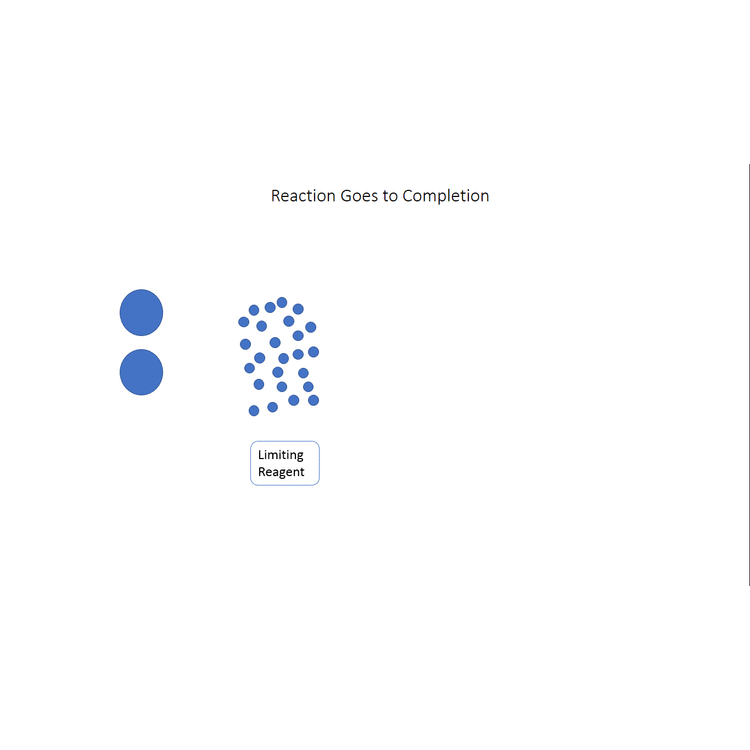# 4.4: Percent Yield

$$\newcommand{\vecs}{\overset { \rightharpoonup} {\mathbf{#1}} }$$ $$\newcommand{\vecd}{\overset{-\!-\!\rightharpoonup}{\vphantom{a}\smash {#1}}}$$$$\newcommand{\id}{\mathrm{id}}$$ $$\newcommand{\Span}{\mathrm{span}}$$ $$\newcommand{\kernel}{\mathrm{null}\,}$$ $$\newcommand{\range}{\mathrm{range}\,}$$ $$\newcommand{\RealPart}{\mathrm{Re}}$$ $$\newcommand{\ImaginaryPart}{\mathrm{Im}}$$ $$\newcommand{\Argument}{\mathrm{Arg}}$$ $$\newcommand{\norm}{\| #1 \|}$$ $$\newcommand{\inner}{\langle #1, #2 \rangle}$$ $$\newcommand{\Span}{\mathrm{span}}$$ $$\newcommand{\id}{\mathrm{id}}$$ $$\newcommand{\Span}{\mathrm{span}}$$ $$\newcommand{\kernel}{\mathrm{null}\,}$$ $$\newcommand{\range}{\mathrm{range}\,}$$ $$\newcommand{\RealPart}{\mathrm{Re}}$$ $$\newcommand{\ImaginaryPart}{\mathrm{Im}}$$ $$\newcommand{\Argument}{\mathrm{Arg}}$$ $$\newcommand{\norm}{\| #1 \|}$$ $$\newcommand{\inner}{\langle #1, #2 \rangle}$$ $$\newcommand{\Span}{\mathrm{span}}$$$$\newcommand{\AA}{\unicode[.8,0]{x212B}}$$Not all chemical reactions are as simple as the ones we have considered, so far. Quite often a mixture of two or more products containing the same element is formed. For example, when octane (or gasoline in general) burns in an excess of air, the reaction is

$2 \text{C}_{8} \text{H}_{18} + 25 \text{O}_{2} \rightarrow 16 \text{CO}_{2} + 18 \text{H}_{2} \text{O}\label{1}$

If oxygen is the limiting reagent, however, the reaction does not necessarily stop short of consuming all the octane available. Instead, some carbon monoxide (CO) forms:

$2 \text{C}_{8} \text{H}_{18} + {24} \text{O}_{2} \rightarrow 14 \text{CO}_{2} + {\text{2CO}}+ 18 \text{H}_{2} \text{O} \nonumber$

Burning gasoline in an automobile engine, where the supply of oxygen is not always as great as that demanded by the stoichiometric ratio, often produces carbon monoxide, a poisonous substance and a major source of air pollution.

In other cases, even though none of the reactants is completely consumed, no further increase in the amounts of the products occurs. We say that such a reaction does not go to completion. When a mixture of products is produced or a reaction does not go to completion, the effectiveness of the reaction is usually evaluated in terms of percent yield of the desired product. A theoretical yield is calculated by assuming that all the limiting reagent is converted to product. The experimentally determined mass of product is then compared to the theoretical yield and expressed as a percentage:

$\text{Percent yield}=\frac{\text{actual yield}}{\text{theoretical yield}}\times \text{100 percent} \nonumber$

The video below (modeled after the octane example given earlier in the chapter) demonstrates visually what the percent yield is, first showing the theoretical yield, then showing the actual yield (where the reaction doesn't go to completion) and finally comparing the actual yield to the theoretical yield to find the percent yield.##### Example $$\PageIndex{1}$$ : Percent Yield

When 100.0 g N2 gas and 25.0 g H2 gas are mixed at 350°C and a high pressure, they react to form 28.96 g NH3 (ammonia) gas. Calculate the percent yield.

Solution:

We must calculate the theoretical yield of NH3, and to do this, we must first discover whether N2 or H2 is the limiting reagent. For the balanced equation

$\ce{N2 + 3H2 -> 2NH3} \nonumber$

the stoichiometric ratio of the reactants is

$\text{S}\left( \frac{\ce{H2}}{\ce{N2}} \right)= \frac{\text{3 mol H}_2}{\text{1 mol N}_2} \nonumber$

Now, the initial amounts of the two reagents are

and

\begin{align} & n_{\text{H}_{\text{2}}}\text{(initial)}&=\text{25}\text{.0 g H}_{\text{2}}\times \frac{\text{1 mol H}_{\text{2}}}{\text{2}\text{.016 g H}_{\text{2}}}=\text{12}\text{.4 mol H}_{\text{2}} \\ & \\ & n_{\text{N}_{\text{2}}}\text{(initial)}&=\text{100}\text{.0 g N}_{\text{2}}\times \frac{\text{1 mol N}_{\text{2}}}{\text{28}\text{.02 g N}_{\text{2}}}=\text{3}\text{.569 mol N}_{\text{2}} \\ \end{align}

The ratio of initial amounts is thus

$\frac{n_{\text{H}_{\text{2}}}\text{(initial)}}{n_{\text{N}_{\text{2}}}\text{(initial)}}=\frac{\text{12}\text{.4 mol H}_{\text{2}}}{\text{3}\text{.569 mol N}_{\text{2}}}=\frac{\text{3}\text{.47 mol H}_{\text{2}}}{\text{1 mol N}_{\text{2}}} \nonumber$

Since this ratio is greater than $$\text{S}\left( \frac{\text{H}_{\text{2}}}{\text{N}_{\text{2}}} \right)$$, there is an excess of H2. N2 is the limiting reagent. Accordingly we must use 3.569 mol N2 (rather than 12.4 mol H2) to calculate the theoretical yield of NH3. We then have

$n_{\text{NH}_{\text{3}}}\text{(theoretical)}=\text{3}\text{.569 mol N}_{\text{2}}\times \frac{\text{2 mol NH}_{\text{3}}}{\text{1 mol N}_{\text{2}}} =\text{7}\text{.138 mol NH}_{\text{3}} \nonumber$

so that

$m_{\text{NH}_{\text{3}}}\text{(theoretical)}=\text{7}\text{.138 mol NH}_{\text{3}}\times \frac{\text{17}\text{.03 g NH}_{\text{3}}}{\text{1 mol NH}_{\text{3}}} =\text{121}\text{.6 g NH}_{\text{3}} \nonumber$

The percent yield is then

\begin{align} \text{Percent yield} & =\frac{\text{actual yield}}{\text{theoretical yield}} \times \text{100 percent } \\ { } \\ &=\frac{\text{28.96 g}}{\text{121.6 g}}\times \text{100 percent} \\ { } \\ &=\text{23.81 percent} \end{align}

Combination of nitrogen and hydrogen to form ammonia is a classic example of a reaction which does not go to completion. Commercial production of ammonia is accomplished using this reaction in what is called the Haber process. Even at the rather unusual temperatures and pressures used for this industrial synthesis, only about one-quarter of the reactants can be converted to the desired product. This is unfortunate because nearly all nitrogen fertilizers are derived from ammonia and the world has come to rely on them in order to produce enough food for its rapidly increasing population. Ammonia ranks third [after sulfuric acid (H2SO4) and oxygen (O2)] in the list of most-produced chemicals, worldwide. It might rank even higher if the reaction by which it is made went to completion. Certainly ammonia and the food it helps to grow would be less expensive and would require much less energy to produce if this were the case.

This page titled 4.4: Percent Yield is shared under a CC BY-NC-SA 4.0 license and was authored, remixed, and/or curated by Ed Vitz, John W. Moore, Justin Shorb, Xavier Prat-Resina, Tim Wendorff, & Adam Hahn.